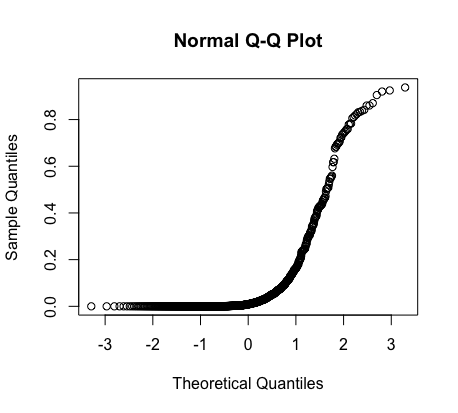1
2
3
4
5
#

Assessing Normality: Normal Probability Plots

Introduction

Statisticians often need tools to assess the normality of data. In other words, they need a way to answer the question: is the data normal or not?

One of the most basic ways to check is by seeing if the Empirical Rule applies. Where x̅ represents the sample mean and s represents the standard deviation, if and only if the data is normal, then:

68% of the data should be between x̅ +/- s

95% of the data should be between x̅ +/- 2s

99.7% of the data should be between x̅ +/- 3s

Another method of assessing normality is creating a normal probability plot.

If the data is normal, then the normal probability plot should be linear.

The Shapes of Normal Probability Plots

 Symmetrical / Normal (No Skew)Skewed RightSkewed LeftHow to Create a Normal Probability Plot (TI-84)

Using a TI-84 calculator, follow these steps to create a normal probability plot:

1. Enter data into calculator by hitting 'STAT' and then 'Edit'

2. Hit '2nd' and then 'Y=' to access Stat Plot.

3. Enter Plot 1.

4. Turn Plot 1 'On' and then toggle below to 'Type.' Then click right until you get to the bottom right graph.

5. Hit 'ZOOM' and then scroll down to '9: Zoom Stat'